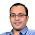## Jan 15, 2013

### Evenly Spaced Ones in Binary String

Source: Sent to me by Piyush Sao (EE IITM Alumnus, Georgia Tech Grad Student)Problem:
Given a arbitrary binary string of length n, find three evenly spaced ones within the string if they exist. Write an algorithm which solves this in O(n * log(n)) time.

Update (29th January 2013):
Solution posted by JDGM in comments!

1.1. Make a list L of positions of 1 in the string. This is O(n).

The problem is now to find an arithmetic progression of length 3 in L.

2. Make a polynomial p with terms xᵏ for each k in L. This is O(n).

3. Find the polynomial q = p² using the Fast Fourier Transform. This is O(n(log n)).

In this new polynomial q, the coefficient of any x²ᵇ for b in L is exactly the number of pairs (a,c) in L such that a+c=2b. One such pair is always (b,b) (with coefficient at least 1), but if there is any other pair (a,c), then the coefficient is at least 3, from (a,c) and (c,a).

4. Scan the coefficients of the terms x²ᵇ looking for values greater than 1. This is O(n).

If none are greater than 1 then there are no evenly spaced 1s. If there is b in L for which the coefficient of x²ᵇ is greater than 1, then we know there is some pair (a,c) for which a+c=2b.

5. Try each a in L (the corresponding c is 2b-a) and check if there is a 1 at position 2b-a in S. This will give our solution. This step is O(n).

2.Great solution. Thanks.

Adding to what you said, the question just asks to find one set of evenly spaced 1s. Hence, even if the number of b's for which the coefficient is > 1 is large, in first scan for one b, you will find the set of 1s that you want to. :)

3.I think this can be done in O(n).

Lets start scanning the bits one by one from the left. I stop When I find the 1st '1'. Let's say I store this index in a variable called a. I continue my search for the next '1'. I find out the distance of the two 1's (subtraction of indices of current and previous '1'). Suppose the distance is coming out to be 'd'. I will just chk whether a+dth bit is '1' or not. If it is '1' I just found a solution. Otherwise I store the index of the current '1' and move on to search the next '1'.

4.I think this can be done in O(n) as follows

let's say i have the bit string in a array str[].

public class EvenlySpacedOnes {

public static void main(String[] args) {
int prev=-1;

String str="";

try {
} catch (IOException e) {
e.printStackTrace();
}

for(int i=0;i<str.length();i++){

if(str.charAt(i)=='1'){
if(prev!=-1){
int next=2*i-prev;
if(next<str.length()){
if(str.charAt(next)=='1'){
System.out.println(prev+" "+i+" "+next);
}
else
prev=i;
}
else
prev=i;
}
else
prev=i;
}
}
}
}

5.This will only find the consecutive ones. The question I guess asks for any set of three ones equally spaced, whether there exists one between them or not.

### Fraction Brainteaser

Source: Sent to me by Gaurav Sinha Problem: Siddhant writes a Maths test and correctly answers 5 out of 6 Arithmetic questions and 20...